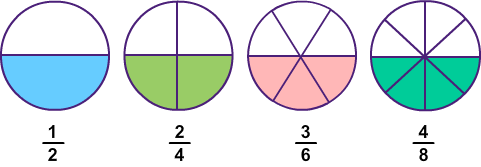Equivalent Fractions
4 years ago
mrchurch
Save
Edit
Host a game
Live GameLive
Homework
Solo Practice
Practice20 QuestionsShow answers
• Question 1
30 seconds
Q. The number on the bottom of a fraction is called what?
numerator
denominator
• Question 2
30 seconds
Q. The number on the top of a fraction is called what?
numerator
denominator
• Question 3
30 seconds
Q. What is an equivalent fraction for 4/4?
4/1
2/2
5/6
1/4
• Question 4
30 secondsQ. All of the fractions in the models are equivalent fractions except...
2/4
4/16
8/16
1/2
• Question 5
30 seconds
Q. Which fraction is equivalent to 1?
1/2
1/10
5/5
5/1
• Question 6
30 seconds
Q. Which is NOT an equivalent fraction to one half?
2/4
2/3
4/8
3/6
• Question 7
30 secondsQ. The model in the picture represents 2/8. Which model represents a fraction equivalent to 2/8?
A
B
C
D
• Question 8
30 secondsQ. What fraction of the rectangle is blue?
1/6
1/3
6/2
2/3
• Question 9
30 secondsQ. Which picture shows 2/8?
green
blue
purple
pink
• Question 10
30 secondsQ. Which picture shows 1/2?
green
blue
purple
pink
• Question 11
30 secondsQ. What fraction is green?
1/4
1/2
2/3
2/3
• Question 12
30 secondsQ. What fraction is red?
1/2
1/3
4/6
2/4
• Question 13
30 secondsQ. What fraction is red?
2/8
2/4
3/4
4/4
• Question 14
300 seconds
Q. Select the equivalent fraction for 2/6
1/4
1/3
1/8
1/2
• Question 15
60 seconds
Q. James thinks 1/2 is equivalent to 4/8.  Is James correct?
Yes, because 4/8 = 1/2
No, because 4/8 = 1/4
• Question 16
60 secondsQ. Use the picture. All of the following are equivalent fractions except ...
1/3
2/6
2/12
3/9
• Question 17
30 secondsQ. All of the following are equivalent fractions except......
1/2
3/12
2/8
4/12
• Question 18
30 secondsQ. What fraction of the circle is pizza?
1/3
2/8
1/2
2/3
• Question 19
30 secondsQ. Use the picture. Which letter represents 2/6?
X
G
C
B
• Question 20
30 seconds
Q. What do you call a fraction that is equal ?